### MATRICES II

Mathematics SSS 3 First Term

WEEK 2

MATRICES II

Performance Objectives

Student should be able to:

1. Perform matrix multiplication of 2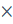2 and 33 matrices.
2. Find the transpose of a matrix.
3. Calculate the determinant of matrices.
4. Solution to simultaneous equation using determinant method.
5. Inverse of 22 matrix.

Content

Let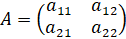and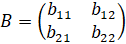The product of the matrices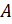and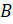wriiten as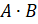or.... View More